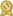# Mr. Casey W.

## specializes in Mathematics (and Science) Instruction by a Mathematician! · male

"Casey is a mathematician, holding a BS in discrete mathematics focusing on computer science and business from the Georgia Institute of Technology, summa cum laude. He was trained and served as an undergraduate teaching assistant for both the Math and Computer Science Departments. Research interests include game theory, network theory, combinatorics, algebra, industrial systems engineering (optimization and more...

"Casey is a mathematician, holding a BS in discrete mathematics focusing on computer science and business from the Georgia Institute of Technology, summa cum laude. He was trained and served as an undergraduate teaching assistant for both the Math and Computer Science Departments. Research interests include game theory, network theory, combinatorics, algebra, industrial systems engineering (optimization and efficiency science), computer science and algorithm design. Casey also holds an MS in Pure Mathematics from the University of Arizona. He held titles of both Graduate Teaching Assistant, serving as lead instructor for many mathematics courses, and Graduate Research Assistant, where research topics covered random matrices, asymptotic analysis, combinatorics, poker modeling and game theory.

Casey now pursues interests in the field of Mathematics Education including:

Early education, focusing on the Common Core mathematics curriculum.

Teaching the fundamental principals of algebra through Arithmetic instruction by using simplified visual learning examples that can be easily grasped by the youngest of learners.

Test prep at all levels including: all mathematics AP exams, SAT, ACT, GRE mathematics, both general and subject specific, LSAT, and science subject tests, like SATII Math and Physics.

Topics I enjoy particularly enjoy teaching include:

Geometry, Algebra, Precalculus, Calculus, Differential Equations, Linear Algebra, Statistics, Mathematical Modeling, Combinatorics, Algorithms, Probability, Logic, Set Theory, Number Theory, Abstract Algebra, and Problem Solving.

Casey has over 15 years experience teaching mathematics at the College and University levels, Elementary, and tutoring Elementary, Middle, and High School students of all ages and varying levels of mathematics knowledge.

From the basics of Arithmetic to advanced Abstract Algebra, the approach to interpreting a problem and solving it never changes. Reformulation of a problem into a context that makes it of inter" less...

# Details

 fee: \$90 (for 60 min) travel distance: 30 miles meeting type: either in-person or online

# Contact

 website: on file

# Education and Qualifications

 certified:Gerogia Institute of Technology (Georgia Tech), Discrete Mathematics and Business

The University of Arizona, Masters

The University of Arizona, PhD

# Subjects Tutored

Algebra 1 - Algebra 2 - Calculus - Geometry - Prealgebra - Precalculus - Trigonometry - Statistics - SAT Math - Probability - PSAT - Elementary Math - Discrete Math - Differential Equations - Computer Science - Linear Algebra - Logic - Finite Math - Common Core